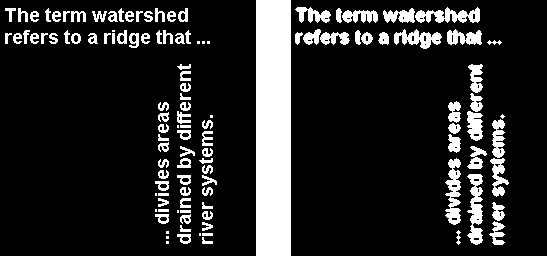## Lookup Table Operations

### Creating a Lookup Table

Certain binary image operations can be implemented most easily through lookup tables. A lookup table is a column vector in which each element represents the value to return for one possible combination of pixels in a neighborhood. To create lookup tables for various operations, use the `makelut` function. `makelut` creates lookup tables for 2-by-2 and 3-by-3 neighborhoods. The following figure illustrates these types of neighborhoods. Each neighborhood pixel is indicated by an x, and the center pixel is the one with a circle.For a 2-by-2 neighborhood, there are 16 possible permutations of the pixels in the neighborhood. Therefore, the lookup table for this operation is a 16-element vector. For a 3-by-3 neighborhood, there are 512 permutations, so the lookup table is a 512-element vector.

Note

`makelut` and `applylut` support only 2-by-2 and 3-by-3 neighborhoods. Lookup tables larger than 3-by-3 neighborhoods are not practical. For example, a lookup table for a 4-by-4 neighborhood would have 65,536 entries.

### Using a Lookup Table

Once you create a lookup table, you can use it to perform the desired operation by using the `applylut` function.

The example below illustrates using lookup table operations to modify an image containing text. The example creates an anonymous function that returns 1 if three or more pixels in the 3-by-3 neighborhood are 1; otherwise, it returns `0`. The example then calls `makelut`, passing in this function as the first argument, and using the second argument to specify a 3-by-3 lookup table.

```f = @(x) sum(x(:)) >= 3; lut = makelut(f,3);```

`lut` is returned as a 512-element vector of 1's and 0's. Each value is the output from the function for one of the 512 possible permutations.

You then perform the operation using `applylut`.

```BW1 = imread('text.png'); BW2 = applylut(BW1,lut); imshow(BW1) figure, imshow(BW2)```

Image Before and After Applying Lookup Table OperationFor information about how `applylut` maps pixel combinations in the image to entries in the lookup table, see the reference page for `applylut`.